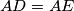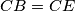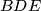### MEMO 2010 pojedinačno problem 3

Kvaliteta:
Avg: 3.0
Težina:
Avg: 5.3
We are given a cyclic quadrilateral$ABCD$ with a point$E$ on the diagonal$AC$ such that$AD=AE$ and$CB=CE$. Let$M$ be the center of the circumcircle$k$ of the triangle$BDE$. The circle$k$ intersects the line$AC$ in the points$E$ and$F$. Prove that the lines$FM$,$AD$ and$BC$ meet at one point.
Source: Srednjoeuropska matematička olimpijada 2010, pojedinačno natjecanje, problem 3